# Weight Chapter 42 Weight The weight of an

• Slides: 7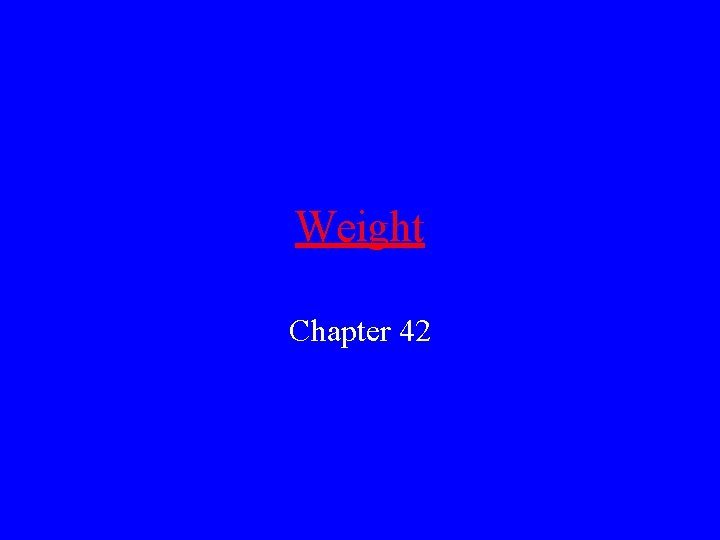Weight Chapter 42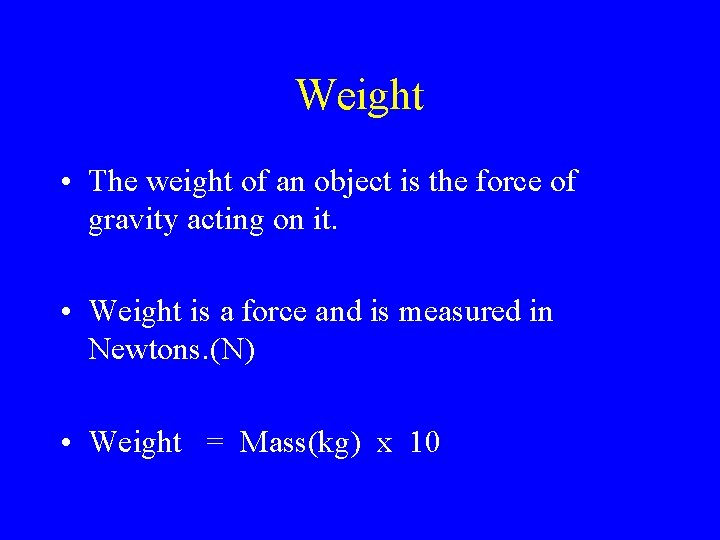Weight • The weight of an object is the force of gravity acting on it. • Weight is a force and is measured in Newtons. (N) • Weight = Mass(kg) x 101 s Speed = 10 m/s 2 s Speed =20 m/s 3 s Speed=30 m/s 4 s Speed=40 m/s Gravity • Weight is the gravitational pull of the earth on an object. • In the diagram the speed of the object is increasing as it falls. • Acceleration due to gravity = 10 m/s/s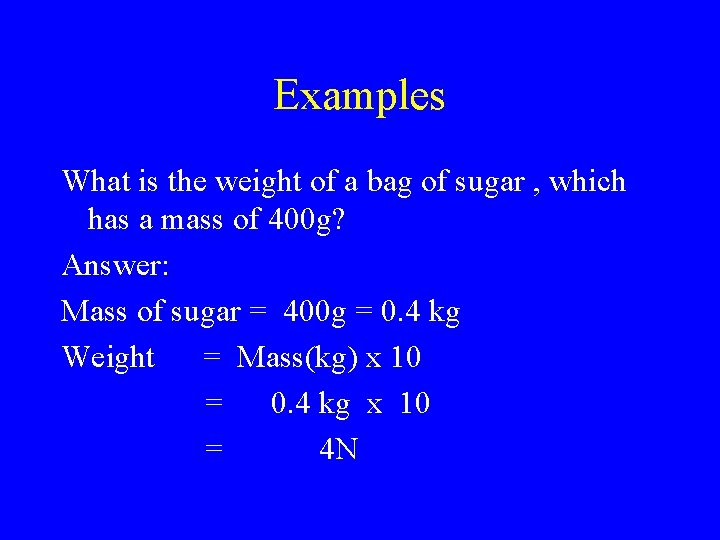Examples What is the weight of a bag of sugar , which has a mass of 400 g? Answer: Mass of sugar = 400 g = 0. 4 kg Weight = Mass(kg) x 10 = 0. 4 kg x 10 = 4 N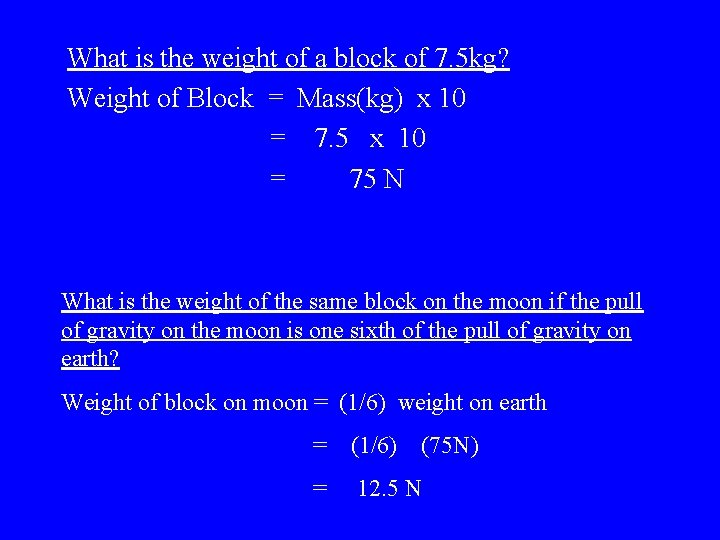What is the weight of a block of 7. 5 kg? Weight of Block = Mass(kg) x 10 = 7. 5 x 10 = 75 N What is the weight of the same block on the moon if the pull of gravity on the moon is one sixth of the pull of gravity on earth? Weight of block on moon = (1/6) weight on earth = (1/6) (75 N) = 12. 5 N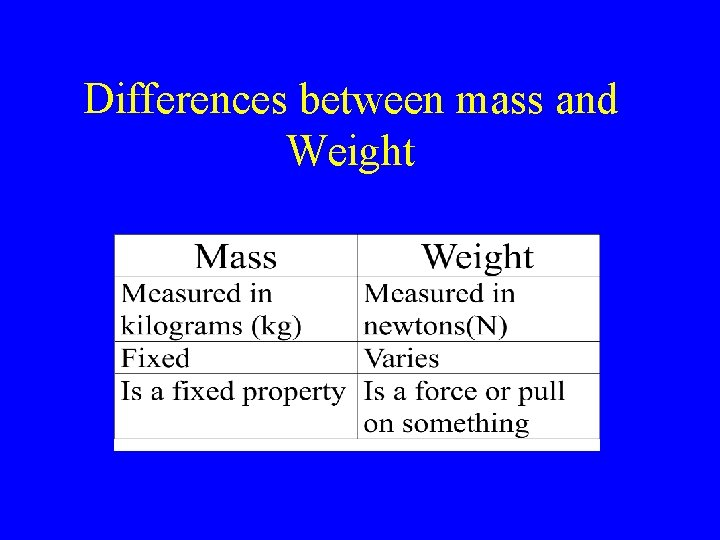Differences between mass and Weight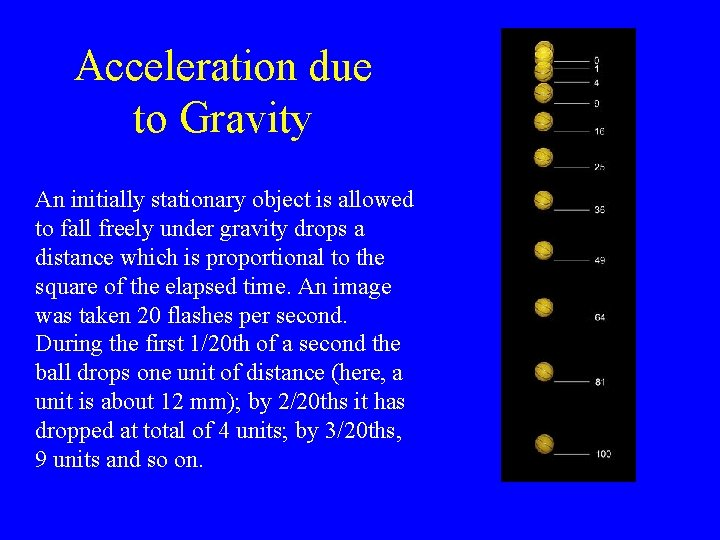Acceleration due to Gravity An initially stationary object is allowed to fall freely under gravity drops a distance which is proportional to the square of the elapsed time. An image was taken 20 flashes per second. During the first 1/20 th of a second the ball drops one unit of distance (here, a unit is about 12 mm); by 2/20 ths it has dropped at total of 4 units; by 3/20 ths, 9 units and so on.